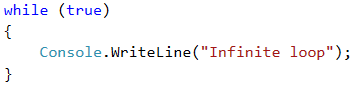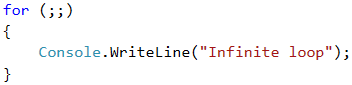# Infinite Loops with Break

So far, we were introduced to various types of loops, learning what structures they have and how they are applied. Now, we need to understand what an infinite loop is, when it occurs, and how we can break it using the break operator.

## Video: Infinite Loops with Break

Watch this video lesson to learn how to use infinite loops, along with the break operator: https://youtu.be/rpez6b9TpdA.

## Infinite Loop – Explanation

We call an infinite loop one that repeats infinitely the performance of its body. In while and do-while loops the end check is a conditional expression that always returns true. Infinite for occurs when there is no condition to end the loop.

Here is what an infinite while loop looks like:And here is what an infinite for loop looks like:## The Operator "Break"

We already know that the infinite loop performs a certain code infinitely, but what if we want at some point under a given condition to go out of the loop? The break operator comes in handy in this situation.The break operator stops the execution of a loop at the time it is called and continues from the first line after the end of the loop. This means that the current iteration of the loop will not be completed, accordingly, the rest of the code in the body of the loop will not be executed.